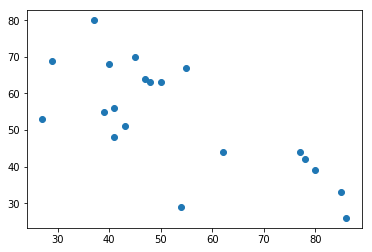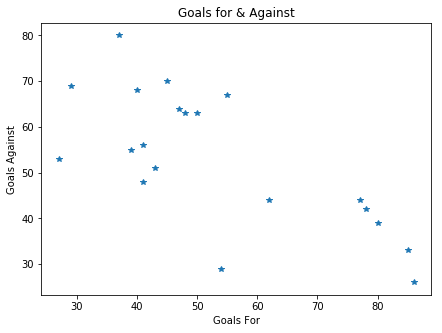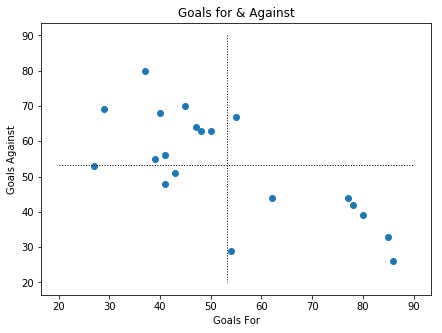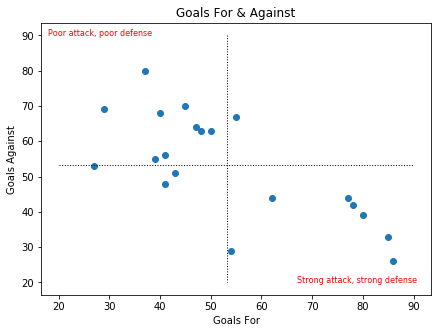# Scatter Plots & Crosshairs in Matplotlib

Across Python’s many visualisation libraries, you will find several ways to create scatter plots. Matplotlib, being one of the fundamental visualisation libraries, offers perhaps the simplest way to do so. In one line, we will be able to create scatter plots that show the relationship between two variables. It also offers easy ways to customise these charts, through adding crosshairs, text, colour and more.

This article will plot goals for and against from a season, taking you through the initial creation of the chart, then some customisation that Matplotlib offers. Import the modules and data and off we go.

In :
```import numpy as np
import pandas as pd
import matplotlib.pyplot as plt
%matplotlib inline
```
In :
```table = pd.read_csv("../../Data/1617table.csv")
```
Out:
Pos Team Pld W D L GF GA GD Pts
0 1 Chelsea 38 30 3 5 85 33 52 93
1 2 Tottenham Hotspur 38 26 8 4 86 26 60 86
2 3 Manchester City 38 23 9 6 80 39 41 78
3 4 Liverpool 38 22 10 6 78 42 36 76
4 5 Arsenal 38 23 6 9 77 44 33 75

Nothing exceptional about our table here. We have exactly the data that we would expect and we are going to plot goals for (GF) and goals against (GA).

Matplotlib’s ‘.plot()’ will make this incredibly easy. We just need to pass it three arguments: the data to plot along each of the axes and the plot type. In this case, the plot type is ‘o’ to show that we want to plot markers. Let’s see what the default chart looks like:

In :
```plt.plot(table['GF'],table['GA'],"o")
```
Out:
`[<matplotlib.lines.Line2D at 0x2488736de10>]`It is quite plain and has no labels, but you can see just how easy it is to do. It is almost just as easy to add some tiles.

Rather than directly plot the chart, we can create our chart area with the first line below, set its size, then add our features from there. Take a look:

In :
```#Create plot area
fig, ax = plt.subplots()

#Set plot size
fig.set_size_inches(7, 5)

#Plot chart as above, but change the plot type from 'o' to '*' - givng us stars!
plt.plot(table['GF'],table['GA'],"*")

ax.set_title("Goals for & Against")
ax.set_xlabel("Goals For")
ax.set_ylabel("Goals Against")

#Display the chart
plt.show()
```Great work! Just a few lines of code make a massive difference to our charts.

This time, let’s add a crosshair to our chart to display the average line. This should help our viewers to see if a point is performing well or not.

To do this, we can use ‘plt.plot()’ again. Once again, we give it 3 arguments as a minimum:

• Type – ‘k-‘ gives us two instructions to plot with. K means black, – means draw a line
• Start/End X locations – Give these two coordinates in a list. In the example below, we calculate the average to get the coordinate.
• Start/End Y locations – Once again, give these coordinates in a list. Below they are [90,20]

We also give two optional arguments. Linestyle changes the line, in this case “:” gives us a dotted line. Meanwhile, lw dictates the line width.

In :
```fig, ax = plt.subplots()
fig.set_size_inches(7, 5)

plt.plot(table['GF'],table['GA'],"o")
plt.plot([table['GF'].mean(),table['GF'].mean()],[90,20],'k-', linestyle = ":", lw=1)
plt.plot([20,90],[table['GA'].mean(),table['GA'].mean()],'k-', linestyle = ":", lw=1)

ax.set_title("Goals for & Against")
ax.set_xlabel("Goals For")
ax.set_ylabel("Goals Against")

plt.show()
```In our chart above, the crosshairs show the averages and it helps us to group teams accordingly. You may want to classify these quadrants with text on the chart and we add this in a similar way to titles.

Rather than ‘.set_title()’, we instead use ‘.text()’. You must give arguments for the x and y location, in addition to the text that you want to write. Our examples below also give information on the colour and size of the text. Take a look at how this comes up:

In :
```fig, ax = plt.subplots()
fig.set_size_inches(7, 5)

plt.plot(table['GF'],table['GA'],"o")
plt.plot([table['GF'].mean(),table['GF'].mean()],[90,20],'k-', linestyle = ":", lw=1)
plt.plot([20,90],[table['GA'].mean(),table['GA'].mean()],'k-', linestyle = ":", lw=1)

ax.set_title("Goals For & Against")
ax.set_xlabel("Goals For")
ax.set_ylabel("Goals Against")

ax.text(18,90,"Poor attack, poor defense",color="red",size="8")
ax.text(67,20,"Strong attack, strong defense",color="red",size="8")

plt.show()
```### Summary

Head back and compare this last chart with the first one. Not only is the most recent one much, much better looking, it also is much more informative. Simple titles tell us what we are looking at, while crosshairs and text give insight.

This article illustrated the versatility of matplotlib and ‘.plot()’ being able to quickly draw charts and add detail to them. You can see above how we set up a chart area, than draw our chart and additional features. Take a look at the documentation for all of the customisations that you can add with matplotlib.

Create your own scatter plots and crosshair features, and check out some of the other visualisation options Matplotlib offers.